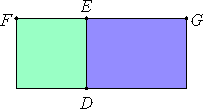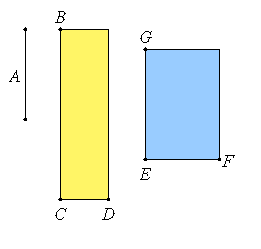# Lemma

If there are two straight lines, then the first is to the second as the square on the first is to the rectangle contained by the two straight lines.

Let FE and EG be two straight lines.I say that FE is to EG as the square on FE is to the rectangle FE by EG.

Describe the square DF on FE, and complete GD.

VI.1

Since then FE is to EG as FD is to DG, and FD is the square on FE, and DG the rectangle DE by EG, that is, the rectangle FE by EG, therefore FE is to EG as the square on FE is to the rectangle FE by EG. Similarly the rectangle GE by EF is to the square on EF, that is GD is to FD, as GE is to EF.

Q.E.D.

# Proposition 22

The square on a medial straight line, if applied to a rational straight line, produces as breadth a straight line rational and incommensurable in length with that to which it is applied.

Let A be medial and CB rational, and let a rectangular area BD equal to the square on A be applied to BC, producing CD as breadth.

I say that CD is rational and incommensurable in length with CB.

X.21

Since A is medial, the square on it equals a rectangular area contained by rational straight lines commensurable in square only.Let the square on it equal GF. But the square on it also equals BD, therefore BD equals GF.

VI.14

But it is also equiangular with it, and in equal and equiangular parallelograms the sides about the equal angles are reciprocally proportional, therefore, BC is to EG as EF is to CD.

VI.22

Therefore the square on BC is to the square on EG as the square on EF is to the square on CD.

X.11

But the square on CB is commensurable with the square on EG, for each of these straight lines is rational, therefore the square on EF is also commensurable with the square on CD.

X.Def.I.4

But the square on EF is rational, therefore the square on CD is also rational. Therefore CD is rational.

And since EF is incommensurable in length with EG, for they are commensurable in square only, while EF is to EG as the square on EF is to the rectangle FE by EG, therefore the square on EF is incommensurable with the rectangle FE by EG.

X.13

But the square on CD is commensurable with the square on EF, for the straight lines are rational in square, and the rectangle DC by CB is commensurable with the rectangle FE by EG, for they equal the square on A, therefore the square on CD is incommensurable with the rectangle DC by CB.

But the square on CD is to the rectangle DC by CB as DC is to CB, therefore DC is incommensurable in length with CB.

Therefore CD is rational and incommensurable in length with CB.

Therefore, the square on a medial straight line, if applied to a rational straight line, produces as breadth a straight line rational and incommensurable in length with that to which it is applied.

Q.E.D.

## Guide

Given medial line A and rational line BC, then CD = A2/(BC) is a rational line incommensurable to BC.

To understand this, let a be the length of A (relative to the standard unit length), b the length of BC, and c the length of CD, so that c = a2/b.

We’re given a medial number a (a4 is a rational number but a2 is an irrational number), and b2 is a rational number. Then it follows that c2 is a rational number (being a4/b2), but c/b is an irrational number (being a2/b2). That follows since the product or quotient of two rational numbers is a rational number, but the product or quotient of an irrational number and a rational number is an irrational number.

This proposition is used frequently in Book X starting with the next proposition.